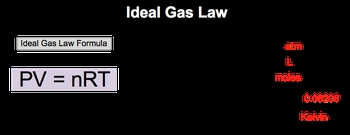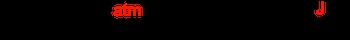## GOB Chemistry

Learn the toughest concepts covered in your GOB - General, Organic, and Biological Chemistry class with step-by-step video tutorials and practice problems.

Gases, Liquids and Solids

# The Ideal Gas Law

The Ideal Gas Law formula relates the behavior of gases under varying conditions of pressure, volume, moles and temperature.

The Ideal Gas Law
1
concept

## The Ideal Gas Law1m
Play a video:
in our exploration and described the behavior of gas is we come upon the ideal gas law. Now the ideal gas law formula relates the behavior of gas under varying conditions, off pressure volume, moles and temperature. So our ideal gas law formula is PV equals NRT. We see that it's in a purple box, which means that you need to memorize it. Your professor is going expect you to know this formula. It's gonna be involved in so many different types of ideas and calculations within this chapter. Now, if we take a closer look at it, we said that P equals pressure here. The units for pressure will be in atmospheres. Volume will be in leaders and equals the amount of gas in moles. Are is a gas constant. And here, when it comes to the ideal gas law, we tend to see it as 0.8 to 06 In the next section, we'll talk about the units involved with this gas constant, and then temperature year temperature will be in Kelvin's. So just remember to talk about the ideal behavior of gas is we use the ideal gas law. So click on the next video and let's take a look at an example question2
example

## The Ideal Gas Law Example 12m
Play a video:
here. We're told that a 500 million container at a pressure of 600 million liters of mercury, possesses 29.3 g nitrogen gas at 50 degrees Celsius. Here, we need to determine the correct units needed for the ideal gas law. So remember, the ideal gas law is PV equals n our teeth. So P stands for pressure. Remember, pressure needs to be in units of atmospheres. So here, we're gonna do a conversion. We're gonna go from 600 millimeters of Mercury into atmospheres millimeters of mercury, go here on the bottom, and then atmosphere go here on top. Remember, the relationship is that for every one atmosphere, it's 760 millimeters of mercury. Okay, so when we do that, we're gonna get a pressure off 0. atmospheres. All those 600 has won six figs. Let's just go with 36 fix here just so that we don't have just 360.8 as atmospheres. Next volume volume needs to be in leaders. So we have 500 mL. And remember, 11 mil e equals 10 to the negative three. So this would just come out as 500 leaders. Next we need moles. So moles is n remember. Nitrogen gas, nitrogen. It's natural or standard State is N two. It's one of our die atomic molecules. So we're told that we have 2029 0.3 g of end to We got to convert that into moles. So one mole of end to so there's two nitrogen. According to the periodic table, each one is 14. g. So multiplying that by two gives me 28.2 g for the combined weight in N o. An end to so grams here, cancel out and I'll have my moles here will put it as 1. moles of end to and then finally, we need our temperature. Ours are constant, so we don't have to worry about giving the correct units is 0. to 06 like we said earlier, So temperature here needs to be in Kelvin. Remember to go from Celsius to Kelvin, you're supposed to add to 73.15. Sometimes professors will just say plus 2, 73. But if you want to be as accurate as possible, you wanna put to 73.15. So when you add that, that's 3. 23.15 Kelvin. So here we have all of the units, um, that we need in the correct forms. So just remember, ideal gas law. And what is the correct unit that we need to utilize for each one of these variables?
3
concept

## The Ideal Gas Law1m
Play a video:
As we said, the ideal gas law uses are, which is our gas constant, but realize that the gas constant are can have two different values, depending on the situation. Now the two terms you'll tend to see when it comes to the are constant is the first one we talked about earlier are being equal 2.8 to 06 will utilize this value when dealing with the ideal gas law, but our can also equal 8.314 This happens when we're dealing with speed, velocity or energy. So if a question is dealing with the speed of an object or a subatomic particle, or dealing with the energy of a reaction or solution through our converts to 8.314 now, the conversion factor between the are groups is that one leader times atmospheres equals one on one point 3 to 5. Jules. So we have our 0.8 to 06 year. We use the conversion factor. So remember we want to get rid of leaders times, atmospheres. So we put them on the bottom. So one leaders times atmosphere here on the bottom is one on 1.3 to 5 jewels here on top leaders. Times atmospheres cancel out, and that's how we end up with jewels at the end. When you plug this in, you get a long string of numbers as 8.314 to 95 jewels over most times K. But we just focus on the 8.314 portion. I know there's a seven there when you work it out completely, but to keep it simple, books will just listed as 8.314 So just keep in mind the gas constant can be two different numbers. And remember what the ideal gas law We use this value, and when dealing with speed, velocity or energy, we use this value of 8.3144
example

## The Ideal Gas Law Example 22m
Play a video:
5
Problem

How many grams of carbon dioxide, CO2, are present in a 0.150 L flask recorded at 525 mmHg and 32 ºC?

a) 1.77 g

b) 0.93 g

c) 0.66 g

d) 0.18 g

e) 0.052 g

6
Problem

How many liters of HNO3 gas, measured at 28.0 ºC and 780 torr, are required to prepare 2.30 L of 4.15 M solution of nitric acid?

7
Problem

When 0.670 g argon is added to a 500 cm3 container with a sample of oxygen gas, the total pressure of the gases is found to be 1.52 atm at a temperature of 340 K. What is the mass of the oxygen gas in the bulb?